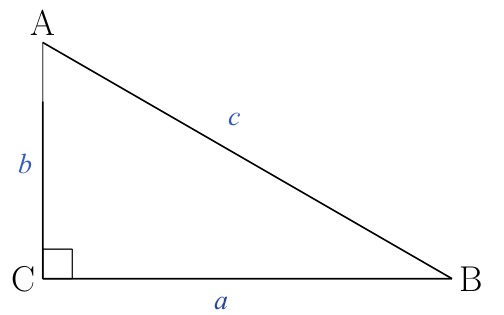# Sine and Cosine

## QuestionIn right triangle ABC, the cosine of angle is 1/2. What is the sine of angle B?

## Solution

The cosine of angle A is the ratio of the length of the side next to angle A (the side that is not the hypotenuse) to the length of the hypotenuse. Using the labelling in the sketch, that’s b/c.

The sine of angle b is the ratio of the length of the side opposite angle  to the length of the hypotenuse. That’s b/c, which, per the question, is 1/2 .

Why was this so easy? The sine of equals the cosine of A. Generally, the sine of an angle equals the cosine of its complement and the cosine of an angle equals the sine of its complement. In a right triangle, the two angles that are not right angles are complementary, so each angle’s sine equals the other angle’s cosine.

For more about sines and cosines and complements, see “Finding an Angle’s Sine Given the Sine of Its Complement.”